# Applying Motion to SimMechanics Models

One of the features I like about SimMechanics is the ability to specify the motion of a system without having to think about the forces involved. This can simplify testing a model so you don't have to design a controller if all you are doing is testing the plant model for correctness. Today I want to talk about a common problem people run into.

The Problem

I have the following SimMechanics model. This model is supposed to simulate a big crane... you know the ones with a big ball at the end of a cable used to destroy buildings.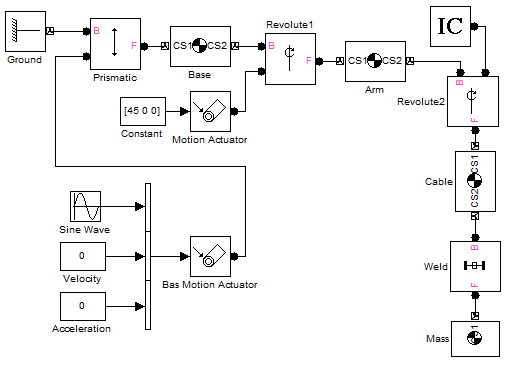When applying a sine wave trajectory (position) to the base, I expect the ball to swing, however it does not!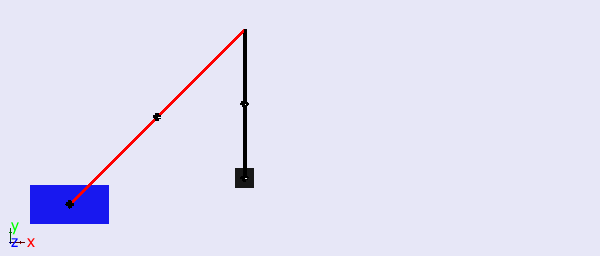The Explanation

As you probably noticed, the base is actuated with a Joint Actuator, setup to Actuate with motion. In this configuration, three components must be fed to the block: Position, Velocity and Acceleration.

Obviously, the velocity and acceleration corresponding to a sine wave trajectory are not 0!

The Solution: First Try

To get valid results with SimMechanics, it is important to provide valid inputs.

To do that, the first thing that probably goes through your mind is to use Derivative blocks, like this: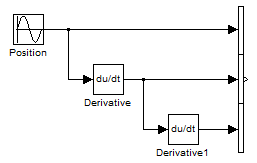This works if the position signal is smooth, however in many cases this can cause problems.

Why? If you have a discontinuity in the position signal, this will cause a sudden change in the velocity signal and an infinite acceleration. Infinite accelerations are not realistic and cause derivative errors in the solver.

A Better Solution

What I recommend is to implement a filtered derivative using a Transfer Function block.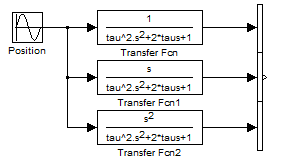Now we can see that our crane behaves as expected.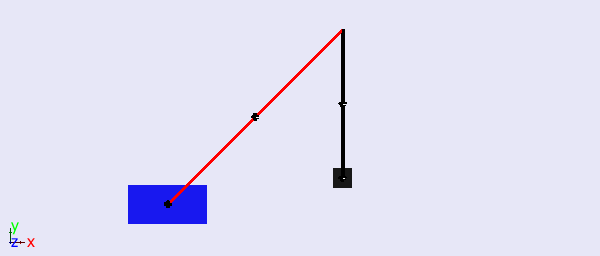Now it's your turn

If you are interested, you can find the model here.

Look at the documentation section titled Stabilizing Numerical Derivatives in Actuation Signals for more details and let us know what you think by leaving a comment here.

|